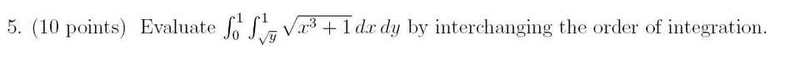# Changing bounds of integration of a double integral

• yopy

#### yopyPicture of the problem is listed above. I'm not sure how to switch the bounds of integration on it. Anyone here know how to go about this?

i tried doing it x^2 to 1 for y and then 0 to 1 for x but it didnt work out to be the write answer, the write answer after putting it in your calculator is about .40 ish

Normally when i do these kinds of problems i draw the bounds out, but I am not sure how these current bounds would even look.

Hi yopy!(try using the X2 tag just above the Reply box)
i tried doing it x^2 to 1 for y and then 0 to 1 for x but it didnt work out to be the write answer …

Well, that looks right to me …

it's just the area above the parabola y = x2

show us what you got.

i figured it out, the bounds turn into 0 to x^2 for respect to Y, and then 0 to 1 for respect to X

my problem was drawing the region they were integrating over. I got the right answer though, thanks.

Hi yopy!(try using the X2 tag just above the Reply box)

Well, that looks right to me …

it's just the area above the parabola y = x2
I don't think so. The limits of integration in the original problem indicate that the region is to the right of the parabola, not above it. The region is bounded by the parabola, the x-axis, and the line x = 1. Yopy's revised limits of integration describe this region.

oops!

I don't think so. The limits of integration in the original problem indicate that the region is to the right of the parabola, not above it. The region is bounded by the parabola, the x-axis, and the line x = 1. Yopy's revised limits of integration describe this region.

oops!i should have drawn it!thanks Mark!